1. 2017.09.11
2. 2016.02.01
3. 2015.12.20
4. 2015.08.31
5. 2015.08.31
6. 2014.08.26
7. 2014.08.09
8. 2014.08.09
9. 2014.08.09
10. 2014.08.09

소프트웨어 기초(Software Foundations)

소프트웨어 기초 (Software Foundations) 번역

- 2017년 9월 대학원 강의로 소프트웨어 기초를 진행하면서 번역한 것을 위 웹 주소에 올려놓았습니다.

[ 교재 ]

New volumes : Vol 1. Logical Foundations, Vol 2. Programming Language Foundations

- https://softwarefoundations.cis.upenn.edu/

[ 강의 로드맵 ]

Vol 1. Logical Foundations : Preface

Vol 1. Logical Foundations : Functional Programming in Coq (Basics)

Vol 1. Logical Foundations : Proof by Induction (Induction)

Vol 1. Logical Foundations : Working with Structured Data (Lists)

Vol 1. Logical Foundations : Polymorphism and Higher-Order Functions (Poly)

Vol 1. Logical Foundations : Logic in Coq (Logic)

Vol 1. Logical Foundations : Inductive Defined Propositions (IndProp)

Vol 1. Logical Foundations : Simple Imperative Programs (Imp)

Vol 2. Programming Language Foundations : Program Equivalence (Equiv)

Vol 2. Programming Language Foundations : Hoare Logic, Part I (Hoare)

[ 전술 목록 :  Ch. More Basic Tactics in Volume 1 ]

• intros: move hypotheses/variables from goal to context
• reflexivity: finish the proof (when the goal looks like e = e)
• apply: prove goal using a hypothesis, lemma, or constructor
• apply... in H: apply a hypothesis, lemma, or constructor to a hypothesis in the context (forward reasoning)
• apply... with...: explicitly specify values for variables that cannot be determined by pattern matching
• simpl: simplify computations in the goal
• simpl in H: ... or a hypothesis
• rewrite: use an equality hypothesis (or lemma) to rewrite the goal
• rewrite ... in H: ... or a hypothesis
• symmetry: changes a goal of the form t=u into u=t
• symmetry in H: changes a hypothesis of the form t=u into u=t
• unfold: replace a defined constant by its right-hand side in the goal
• unfold... in H: ... or a hypothesis
• destruct... as...: case analysis on values of inductively defined types
• destruct... eqn:...: specify the name of an equation to be added to the context, recording the result of the case analysis
• induction... as...: induction on values of inductively defined types
• inversion: reason by injectivity and distinctness of constructors
• assert (H: e) (or assert (e) as H): introduce a "local lemma" e and call it H
• generalize dependent x: move the variable x (and anything else that depends on it) from the context back to an explicit hypothesis in the goal formula

[전술 목록 : Ch.3 from Coq in a Hurry ]

'Coq 증명기' 카테고리의 다른 글

 소프트웨어 기초(Software Foundations)  (0) 2017.09.11 2014.08.26 2014.08.09 2014.08.09 2014.08.09 2014.08.09

인덕션과 코인덕션(Induction & Coinduction)

(아래 내용을 자유롭게 이용하되 다른 웹 사이트 등을 통한 배포를 금합니다.)

인덕션을 사용한 증명은 꽤 익숙하지만, 코인덕션을 사용한 증명은 난해하다. 이 메모를 통해 인덕션과 코인덕션의 원리를 이해해보려 한다.

1. 인덕션과 코인덕션의 수학적 정의 (벤자민 피어스의 <타입과 프로그래밍언어> 책의 21.1절에서)

전체 집합(universal set) U를 가정하자.

[정의] 함수 F \in P(U) -> P(U)가 아래와 같은 성질을 만족하면 단조증가(monotone)한다고 정의한다.

- X \subseteq Y 이면 F(X) \subseteq F(Y)이다.

이후에 언급된 F는 P(U)에 대한 단조증가 함수라고 가정하자. F를 생성함수(generating function)라 부른다.

[정의] X는 U의 부분집합이라고 가정할 때,

- F(X) \subseteq X이면, X는 F에 관해 닫혀있는(F-closed)는 집합이라고 정의하고,

- X \subseteq F(X)이면, X는 F에 관해 일관성이 있는(F-consistent) 집합이라고 정의하며,

- F(X) = X이면, X는 F의 고정점(fixed point)라고 정의한다.

[정리] Knaster-Tarski (Tarski 1955)

- 모든 F 닫힘 집합들의 교집합은 F의 최소 고정점이고,

- 모든 F 일관성 집합들의 합집합은 F의 최대 고정점이다.

[정의] F의 최소 고정점을 \mu F, 최대 고정점을 \nu F라고 정의한다.

[따름 정리]

- 인덕션 원리(Principle of induction) : 만일 X가 F에 관해 닫혀 있다면 \mu F \subseteq X이다.

- 코인덕션 원리(Principle of coinduction) : 만일 X가 F에 관해 일관성이 있다면 X \subseteq \nu F이다.

2. 인덕션 원리의 일반적인 서술과 비교

우리가 아는 인덕션 원리는 보통 이렇게 서술한다. 어떤 술어 명제 P가 존재해서, P(0)가 참이고, 또한 P(i)가 참이면 P(i+1)이 참임을 보일 수 있다면 모든 자연수 i에 대해 P(i)는 참이다. 위의 따름 정리와 연관시켜 설명해본다.

전체 집합 U를 자연수의 집합인 예를 살펴보자. 어떤 명제 P (U의 부분 집합)가 있어서 P(0)이 참이고 P(i)가 참이면 P(i+1)이 참이라고 하자. 생성함수 F를 다음과 같이 정의한다.

- F(X) = { 0 } U { i+1 | i \in X }

F의 정의에 의해 F(P) \subseteq P이다. 왜냐하면,

- F(P)에 0이 포함되어 있는데, P(0)은 참이므로 0 \in P이다.

- 만일 P에 i가 포함되어 있다면 F(P)에 i+1이 포함된다. P(i)가 참이면 P(i+1)도 참이므로 i+1 \in P이다.

이 결과, P는 F에 관해 닫혀있(는 집합이)다. 따름 정리에 의하면

- \mu F \subseteq P

모든 자연수 (\mu F)가 P에 포함되어 있으므로, 인덕션에 의해 모든 자연수 n에 대해 항상 P가 성립함을 보였다.

인덕션 원리에서 주어진 명제가 F에 대해 닫혀있음을 보이는 과정이 우리가 일반적으로 인덕션 원리를 적용해서 증명하는 과정과 일치한다.

3. 코인덕션 원리를 적용한 간단한 증명 예

따름 정리의 코인덕션 원리를 사용하는 증명 방법은

- \nu F (코인덕티브 방식으로 정의한 집합, coinductively defined set)에 어떤 원소 x가 포함되는지를 보이기 위해서

- x를 포함하는 집합 X가 F에 관하여 일관성이 있음으로 보이면 된다.

간단한 오토마타 A = (q0, Q, F, R)을 가정하자. Q는 q0를 포함한 상태 집합, F는  Q의 부분집합으로 최종 상태, R은 Q x Q의 부분집합으로 전이 규칙을 전이한다.

주어진 오토마타가 무한한 전이를 반복할 수 있음을 증명하는 문제를 코인덕션 원리를 가지고 증명해보자. 오토마타의 전이는 다음의 문법으로 생성되는 상태 나열(state sequence)로 표현한다.

Trace ::= q0 | Trace . q

주어진 오토마타 A에 대해, 무한한 전이를 표현하는 생성함수 F를 다음과 같이 정의한다.

- F :: P(Trace) -> P(Trace)

- F(X) = { t . q | t \in X, ( laststate(t), q ) \in R, q \not\in F }

\nu F는 무한한 전이들의 모두 모아놓은 집합이다. (왜냐하면, F 일관성을 갖는 집합을 모두 합해 놓았기 때문이다.)

오토마타 A0 = ( q0, {q0}, {}, { (q0, q0) } )는 직관적으로 무한히 반복되는 전이를 갖고 있다. 이를 코인덕션 원리로 증명한다.

- X를 {  q0 . q0 . ....  }라고 하자. 즉 q0가 무한히 반복되는 원소를 하나 가지고 있는 집합이다.

- X \subseteq F(X)이다. X에 있는 무한 전이 끝에 q0를 붙여도 동일한 무한 전이가 되기 때문이다.

- 집합 X는 F에 관해 일관성이 있으므로, 코인덕션 원리에 의해 X \subseteq \nu F이다.

- \nu F는 A0의 모든 무한 전이를 포함하는 집합이므로 A0가 무한 전이를 포함하고 있음을 증명하였다.

코인덕션 원리로 표현하기 편리한 성질이 있다. 무한한 전이를 포함한다는 것은 이러한 성질의 한가지 사례이다. 인덕션 원리의 경우 X의 원소 수가 무한한 것은 허용하지만, 원소 자체가 무한한 성질을 가지고 있지 못한다. 따라서 이 오토마타 증명 예제의 경우 코인덕션 원리로 증명하는 것이 더 용이하다.

4. 인덕션 원리과 코인덕션 원리의 표현력의 관계

인덕션 원리로 증명할 수 없는 것을 코인덕션 원리로 증명하거나 혹은 반대의 경우가 된는 명제가 있을까? H교수에 의하면 코인덕션 원리로 증명할 수 있는 것은 인덕션 원리로 증명할 수 있다고 한다. 그러나 증명이 복잡해진다고 한다. 이를 간단하게 설명할 수 있을까? 무한히 반복되는 것을 함수로 인코딩해서 표현하는 기법을 응용해야할 수도 있겠다.

[ Help !!! ]

'기타' 카테고리의 다른 글

 인덕션과 코인덕션(Induction & Coinduction)  (0) 2016.02.01

그림으로 설명하는 펑터, 아플리커티브, 모나드

여기 간단한 값(value)의 예가 있다:이 값에 함수를 적용해 보자:간단하지요. 어떤 문맥 안에 값을 놓고 반복해보자. 당분간 문맥을 값을 집어 넣을 수 있는 박스로 생각하자.:함수를 이 값에 적용하면 그 문맥에 따라 다른 결과를 얻을 것이다. 펑터, 어플리커티브, 모나드, 애로우 등 모든 개념이 이 아이디어를 기초로 하고 있다.  Maybe 데이터 타입은 두 가지 연관된 문맥을 정의한다:data Maybe a = Nothing | Just a

Just a와 Nothing일 때 함수를 적용하는 방법이 다르다는 것을 바로 알 수 있을 것다. 먼저 펑터에 대해 얘기하자!

펑터(Functors)

문맥 안에 값을 감싸두면 보통 함수를 그 값에 적용할 수 없다: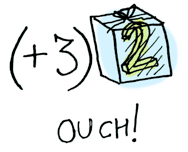이런 상황에서 fmap이 필요하다. fmap은 문맥을 알고 있다. fmap을 통해 문맥 속의 값에 함수를 적용할 수 있다. 함수 (+3)을 Just 2에 적용한다고 가정해보자. fmap을 사용하자:

> fmap (+3) (Just 2)
Just 5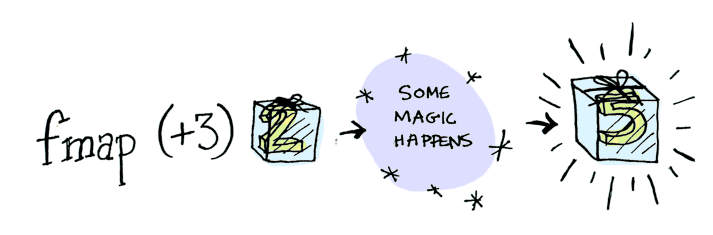두둥!  fmap은 어떻게 하는지 가르쳐준다. 그런데 fmap은 이 함수를 적용하는 법을 어떻게 알 까?

펑터가 뭐지, 진짜?

펑터는 타입클래스다. 여기 그 정의가 있다: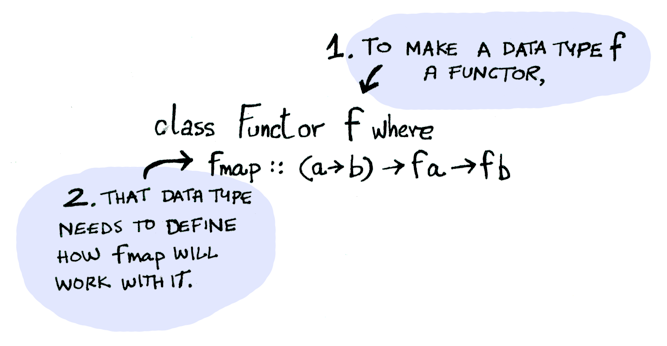Functor는 fmap을 적용하는 방법을 정의하는 데이터타입이다. 다음은 fmap의 동작 방식이다: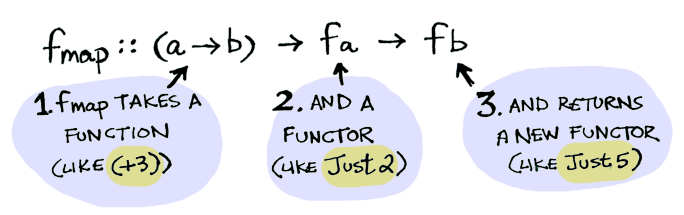따라서 이렇게 할 수 있다:

> fmap (+3) (Just 2)
Just 5

Maybe는 펑터이기 때문에 마법같이 fmap으로 이 함수를 적용한다. 이 펑터로 fmap을 Just들과 Nothing들에 어떻게 함수를 적용하는지 정한다:

instance Functor Maybe where
fmap func (Just val) = Just (func val)
fmap func Nothing = Nothing

fmap (+3) (Just 2)라고 코드의 동작을 다음과 같이 설명할 수 있다: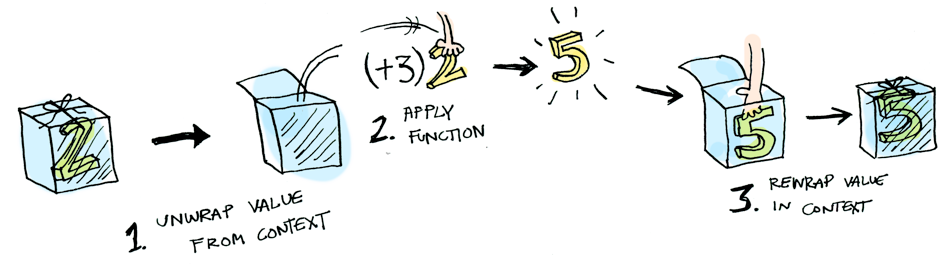fmap으로 (+3)을 Nothing에 적용해볼까?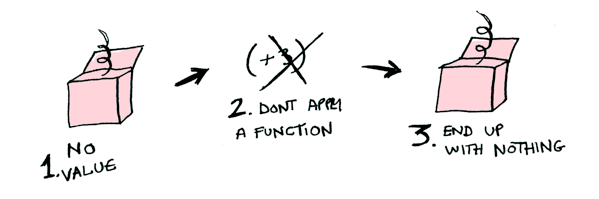> fmap (+3) Nothing
Nothing빌 오라일리, Maybe 펑터에 대해 전혀 알고 있지 않은

영화 매트릭스의 모피어스처럼 fmap은 무엇을 해야할지 알고 있다. Nothing을 가지고 시작하면 Nothing으로 끝날 뿐이다! fmap은 젠(zen)이다. 이제 Maybe 데이터타입이 존재하는 이유가 있다. 예를 들어, Maybe가 없는 언어로 데이터베이스 레코드를 다룬다고 하자:

post = Post.find_by_id(1)
if post
return post.title
else
return nil
end

하스켈로 옮겨보면:

fmap (getPostTitle) (findPost 1)

만일  findPost에서 포스트를 반환하면 getPostTitle로 그 제목을 얻을 수 있다. 만일 findPost에서 아무것도 반환하지 않으면 (Nothing을 반환하면Nothing을 반환할 것이다. 꽤 깔끔하죠? <$>은 fmap의 인픽스(infix) 버전으로, 위 코드 대신 다음과 같이 작성할 수 있다: getPostTitle <$> (findPost 1)

다른 예를 보자: 함수를 리스트에 적용하면 어떻게 되나?리스트도 펑터다! 여기 그 정의가 있다:

instance Functor [] where
fmap = map

오케이, 마지막 예: 함수에 다른 함수를 적용하면 어떨까?

fmap (+3) (+1)

여기 함수가 있다: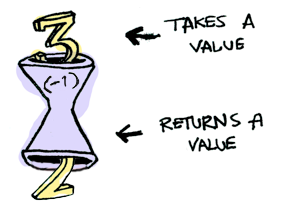여기 다른 함수에 적용할 함수가 있다: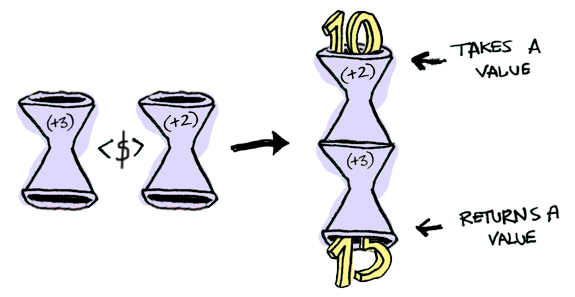그 결과는 단지 또다른 함수다!

> import Control.Applicative
> let foo = fmap (+3) (+2)
> foo 10
15

따라서 함수도 펑터다!

instance Functor ((->) r) where
fmap f g = f . g

fmap을 함수에 사용하면 그 결과는 함수 조합이 된다!

어플리커티브(Applicatives)

어플리커티브는 그 다음 레벨로 데려다준다. 어플리커티브를 사용하면, 펑터처럼, 값을 어떤 문맥 안에 감쌀 수 있다:그러나 함수도 문맥 안에 감쌀 수 있다!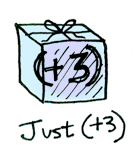자, 이해해보자. Control.Applicative에서 <*>를 두어 어떤 문맥에 감싸져 있는 함수를 관련된 문맥 속에 있는 값에 적용하는 방법을 지정한다: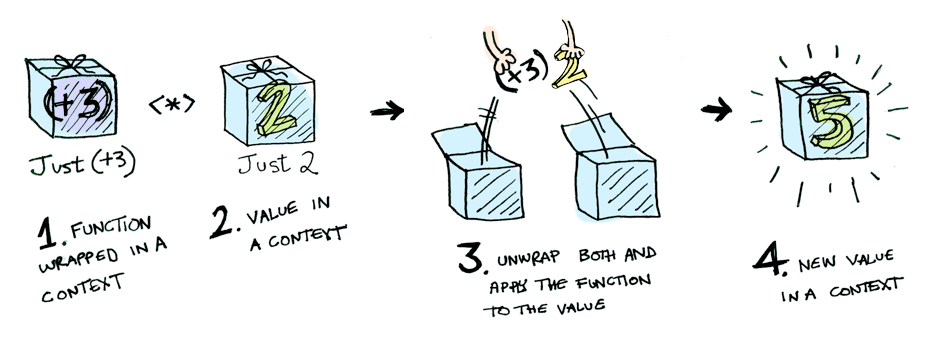예컨데:

Just (+3) <*> Just 2 == Just 5

<*>를 사용하면 재미있는 상황이 벌어질 수 있다. 예를 들어:

> [(*2), (+3)] <*> [1, 2, 3]
[2, 4, 6, 4, 5, 6]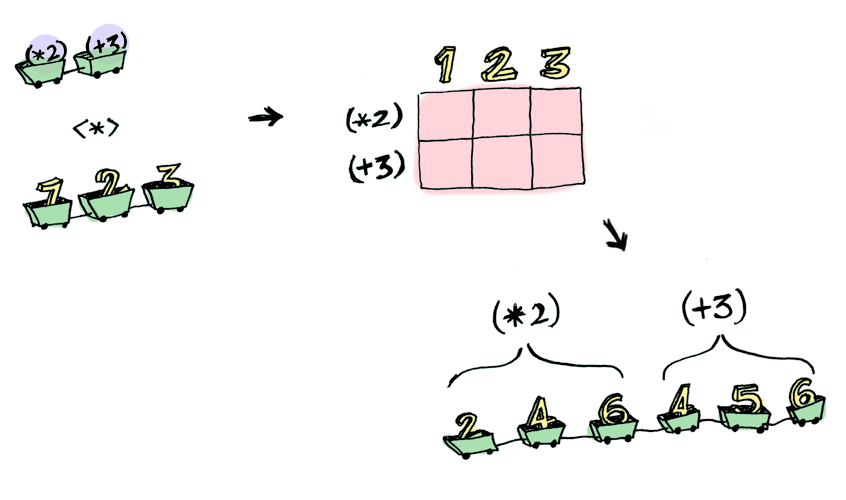펑터로는 할 수 없지만 어플리커티브로 할 수 있는 것이 있다. 두 개의 인자를 받는 함수를 문맥에 감싸져 있는 두 개의 값에 어떻게 적용할 수 있을까?

> (+) <$> (Just 5) Just (+5) > Just (+5) <$> (Just 4)
ERROR ??? WHAT DOES THIS EVEN MEAN WHY IS THE FUNCTION WRAPPED IN A JUST

어플리커티브:

> (+) <$> (Just 5) Just (+5) > Just (+5) <*> (Just 3) Just 8 Applicative는 Functor보다 유연하다. "다 큰 남자는 인자를 몇 개 받든 상관없이 어떠한 함수라도 사용할 수 있어야 한다."라고 마치 말하는 것 같다. "<$>와 <*>를 가지고 임의 개수의 값을 받는 어떤 함수에 포장된 값들을 전달하고 그 결과로 포장된 값을 반환 받을 수 있다!"

> (*) <$> Just 5 <*> Just 3 Just 15 이러한 똑같은 일을 하는 liftA2라 부르는 함수가 있다: > liftA2 (*) (Just 5) (Just 3) Just 15 모나드(Monads) 모나드를 배우는 방법: 1. 컴퓨터 과학 분야의 박사학위를 얻은 다음, 2. 그것을 던져 버린다. 왜냐하면 여기 설명에서는 그 학위가 필요하지 않기 때문에! 모나드는 새로운 방법을 추가한다. 펑터는 함수를 포장된 값에 적용한다: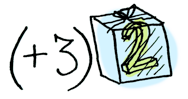어플리커티브는 포장된 함수를 포장된 값에 적용한다: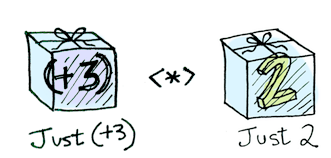모나드는 포장된 값을 반환하는 함수에 포장된 값을 적용한다.모나드는 바인드라고 읽는 함수로 이것을 한다. 예를 살펴보자. Maybe는 모나드다:half는 짝수에 대해 동작하는 함수라 가정하자: half x = if even x then Just (x div 2) else Nothing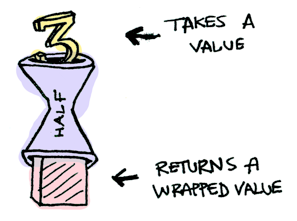포장된 값을 전달하면 무슨 일이 벌어질까?함수 >>=를 사용해서 포장된 값을 함수로 밀어낼 수 있다. 함수 >>=의 사진이 여기 있다:동작 방식을 설명한다: > Just 3 >>= half Nothing > Just 4 >>= half Just 2 > Nothing >>= half Nothing 내부에서 어떤 일이 벌어지고 있나? Monad는 타입클래스다. 부분적으로 아래와 같이 정의한다: class Monad m where (>>=) :: m a -> (a -> m b) -> m b 바인드 함수 >>=는: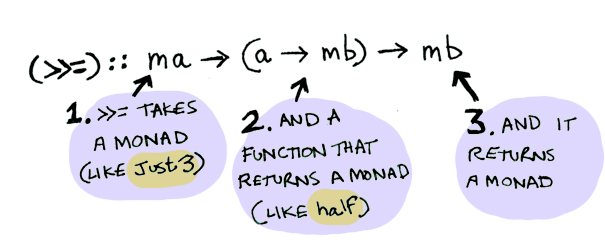따라서 Maybe는 Monad다: instance Monad Maybe where Nothing >>= func = Nothing Just val >>= func = func val 아래에서 Just 3에 대한 동작을 설명한다!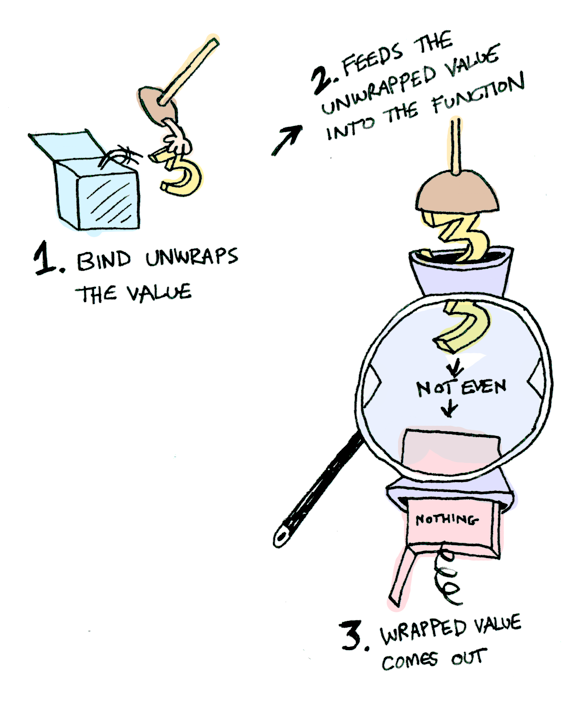Nothing을 주는 경우는 더 간단하다: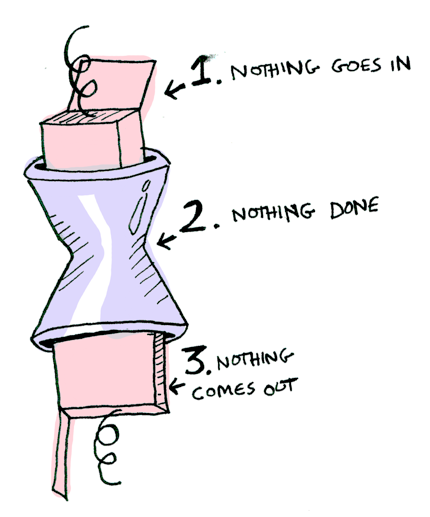이런 함수 호출들을 차례로 묶을 수 있다: > Just 20 >>= half >>= half >>= half Nothing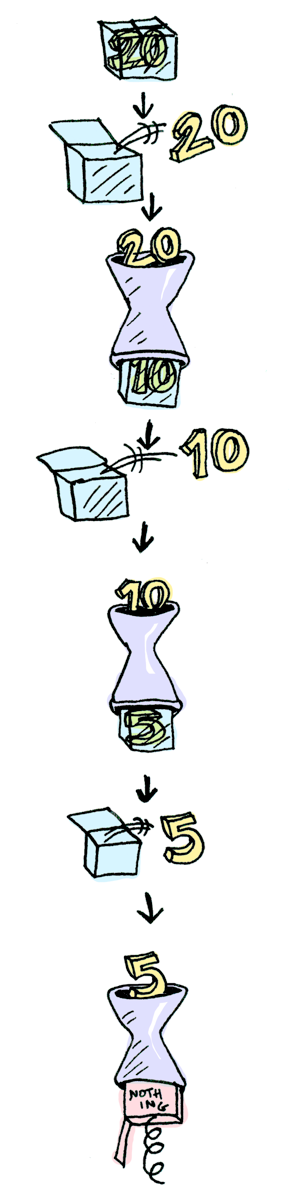쿨! 이제 Maybe는 펑터, 어플리커티브, 모나드라는 것을 알았다. 이제 다른 예를 반복해서 살펴보자: IO 모나드:특히 3가지 함수들. getLine은 특별한 인자 없이 사용자 입력을 받는다:getLine :: IO String readFile은 파일 이름 문자열을 인자로 받고 그 파일 내용을 반환한다:readFile :: FilePath -> IO String putStrLn은 문자열을 받아 출력한다:putStrLn :: String -> IO () 세가지 모든 함수들은 일반 값을 받아(또는 받지 않고) 포장된 값을 반환한다. 이 함수들을 바인드 함수 >>=를 사용하여 차례대로 묶을 수 있다!getLine >>= readFile >>= putStrLn 하스켈 언어는 모나드를 사용하는데 편리한 구문, do 표기법을 제공한다: foo = do filename <- getLine contents <- readFile filename putStrLn contents 결론 1. 펑터는 Functor 타입클래스를 구현하는 데이터 타입이다. 2. 어플리커티브는 Applicative 타입클래스를 구현하는 데이터 타입이다. 3. 모나드는 Monad 타입클래스를 구현하는 데이터 타입이다. 4. Maybe는 이 세가지를 모두 구현한다. 따라서 펑터이고, 어플리커티브이며, 모나드이다. 이 세가지의 차이점은 무엇인가?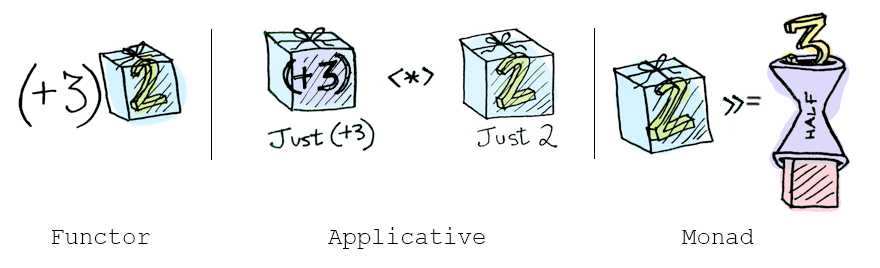• 펑터: fmap이나 <$>를 사용하여 함수를 포장된 값에 적용한다.
• 어플리커티브: <*>나 liftA를 사용하여 포장된 함수를 포장된 값에 적용한다.
• 모나드: >>=나 liftM을 사용하여 포장된 값을 리턴하는 함수를 포장된 값에 적용한다.

자, 여기까지 설명을 들었다면 모나드가 쉽고 SMART IDEA(tm)라는 점에 우리 모두 동의할 것이라 생각한다.  이 가이드로 여러분의 궁금증을 일부 해소했다면 이제 더 심화된 내용을 살펴볼 차례다. LYAH의 모나드 섹션을 살펴보라. 이 가이드에서 넘어갔던 많은 것이 있다. Miran은 자세한 설명을 해줄 것이다.

 그림으로 설명하는 하스켈 펑터, 어플리커티브, 모나트  (1) 2015.12.20 2015.08.31 2015.08.31

댓글을 달아 주세요

1. 먹튀 검증 2018.08.23 10:10 신고

잘보고갑니다~

하스켈 프로그래밍 스터디 계획

첫번째 하스켈 프로그래밍 스터디 계획

펜실베이나 대학(Univ. of Pennsylvania)의 스테파니 웨어릭(Stephanie Weirich) 교수의 CIS 552 고급 프로그래밍 강의 자료를 가지고 하스켈 프로그래밍 스터디를 진행한다.

목차

1. 소개
2. 리스트와 퍼스트 클래스 함수
3. 고차원 프로그래밍 패턴
4. 타입 클래스
5. 모나드 소개
6. 상태 모나드
7. 임의의 모나드와 입출력
8. 모나드를 사용한 파싱 [1,2]
9. 퀵체크(QuickCheck) [1,2]
10. 모나드 변환
11. 동시성을 제공하는 모나드 [1,2]
12. 순수 함수 자료 구조
13. 일반화된 엘지브레익 데이터타입(Generalized Algebraic Datatypes)
14. 타입을 유지하는 추상 구문
15. 타입 리플렉션(Type Reflection) [1,2]

참고 강의 사이트
• http://www.seas.upenn.edu/~cis552/11fa/schedule.html

 그림으로 설명하는 하스켈 펑터, 어플리커티브, 모나트  (1) 2015.12.20 2015.08.31 2015.08.31

댓글을 달아 주세요

1994년 대학원에 들어가서 처음 함수형 프로그래밍 언어 하스켈(Haskell, www.haskell.org)을 접하고 여러 잡다한 프로그래밍을 통해 그 이상한 매력에 끌리게 되었다. 마치 결벽증 환자처럼 "순수함"을 유지하려고 애쓰는 모습도 희한했고, 그러면서도 남들이 하는 일을, 똑같이, 조금 과장해서 말하자면, 더 잘 할 수 있는 것도 멋있었다. 그 매력에 끌려 한때는 모든 다른 프로그래밍 언어는 삼류고, 다 필요없고, 오직 하스켈만 절대 지존이고 유일한 프로그래밍 언어라고 생각했던 적도 있었다. 물론 착각이고.

20년이 지난 오늘 주변에서 하스켈에 대해 관심있는 사람들이 몇몇 있다. 아직은 학교에 있는 학생들이 대부분인 것 같고 L모 회사에 근무하는 사람도 관심을 가지고 있더라. 그리고 몇 주 전에 참가한 어느 세미나에서 "모나드(Monad)"에 대한 설명을 듣는데 개인적으로 너무 마음에 들지 않았다. 하스켈의 악명 높은 주제이긴 하지만 다른 식으로 얘기할 수 있지 않았을까 생각했다. 하스켈이 유일 무이한 프로그래밍 언어는 아니라는 것을 잘 알고 있지만, "순수함"을 잃지 않으려는 이 대범한 노력을 누가 또 할 수 있을까 생각할 때마다 하스켈 프로그래밍을 하던 학생 시절에 그랬던 것 처럼 가슴이 뛰기도 한다. 그리고 "순수함"을 유지하는 하스켈에 "순수함"을 잃어버린 다른 프로그래밍 언어들이 지향해야하는 모습이 담겨 있다는 생각까지 하게 되면, 하스켈 프로그래밍을 배우는 것은 단지 하스켈 언어만을 배우는 것이 아니라는 생각이 강하게 든다.

이러한 생각을 하다보니 하스켈 프로그래밍 커뮤니티가 하나쯤은 있어도 되겠다는 생각이 문득 들었다. 먼저, 하스켈에서 배운대로, 특별한 개발 목적이 있어서가 아니라 그냥 재미삼아 하스켈 언어를 배우는 스터디 모임을 가지고 커뮤니티를 시작해보려 한다. 이 커뮤니티가 활성화되면 하스켈에 적용된 최신 프로그래밍언어 기술에 대해서 논의하고, 나아가서 상용 목적으로 하스켈을 응용하는 사례에 대해 살펴보는 장으로 발전하기를 기대해본다.

2015/8/31

K. Choi

 그림으로 설명하는 하스켈 펑터, 어플리커티브, 모나트  (1) 2015.12.20 2015.08.31 2015.08.31

타입이 다른 원소들의 리스트 (Heterogeneous Lists)

아담 칠리파라(Adam Chlipala)의 책 9.2절 Heterogeneous Lists 참고.

ML이나 Haskell에서 리스트는 매우 빈번하게 사용한다. [1,2,3,4,5]는 1부터 5까지 정수를 포함하는 리스트로 그 타입은 Haskell에서 [Int]이다. ML이나 Haskell에서의 리스트는 타입이 동일한 원소들의 리스트를 얘기한다. 따라서, [1, true]와 같은 식은 허용하지 않는다. Haskell에서 타입 클래스(type class)를 사용하면 타입이 다른 원소들의 리스트를 표현할 수는 있지만 복잡하다. 콕 시스템은 ML이나 Haskell보다 더 복잡한 타입 시스템을 갖추고 있어 상대적으로 간단하게 이러한 리스트를 표현할 수 있다.

콕 시스템에서 타입이 다른 원소들의 리스트를 만드는 방법을 살펴본다. 기본적인 아이디어는 리스트 타입을 타입 수준의 리스트로 색인을 달아 각 원소의 타입이 무엇인지 설명하는 것이다. 간단한 생각이지만 콕으로 표현했을때 간단한 리스트도 꽤 복잡해진다.

Inductive hlist (A:Type) (B:A ->Type) : list A -> Type :=

HNil : hlist A B nil

| HCons : forall (x:A) (ls:list A), B x -> hlist A B ls -> hlist A B (x::ls).

예를 들어 hlist의 쓰임새를 살펴보자. 먼저, HCons의 타입은 선언된 바와 같다. 전칭 한정 변수가 네 개이다.

Check HCons.

HCons

: forall (A : Type) (B : A -> Type) (x : A) (ls : list A),

B x -> hlist A B ls -> hlist A B (x :: ls)

첫번째 전칭 한정 변수 A를 Set으로 대체한다.

Check HCons Set.

HCons Set

: forall (B : Set -> Type) (x : Set) (ls : list Set),

B x -> hlist Set B ls -> hlist Set B (x :: ls)

두번째 전칭 한정 변수 B를 (fun T: Set => T)로 대체한다. 사실 이 함수는 Set -> Set 타입을 갖는다. 서브 타입 관계에 의해 Set -> Type으로 간주한다.

Check HCons Set (fun T:Set => T).

HCons Set (fun T : Set => T)

: forall (x : Set) (ls : list Set),

(fun T : Set => T) x ->

hlist Set (fun T : Set => T) ls ->

hlist Set (fun T : Set => T) (x :: ls)

그 다음 전칭 한정 변수 x에 bool을 넘긴다. bool의 타입은 Set이다.

Check HCons Set (fun T:Set => T) bool.

HCons Set (fun T : Set => T) bool

: forall ls : list Set,

(fun T : Set => T) bool ->

hlist Set (fun T : Set => T) ls ->

hlist Set (fun T : Set => T) (bool :: ls)

마지막 전칭 한정 변수 ls에 nil을 준다. nil은 list Set 타입이므로 타입 리스트이다.

Check HCons Set (fun T:Set => T) bool nil.

HCons Set (fun T : Set => T) bool nil

: (fun T : Set => T) bool ->

hlist Set (fun T : Set => T) nil ->

hlist Set (fun T : Set => T) (bool :: nil)

(fun T : Set => T) bool을 베타 환원(beta redunction)으로 계산하면 bool이 된다. 따라서 HCons Set (fun T : Set => T) bool nil의 타입은 bool -> ... 형태이다. 그래서 이 함수의 부울 인자로 true를 지정한다.

Check HCons Set (fun T:Set => T) bool nil true.

HCons Set (fun T : Set => T) bool nil true

: hlist Set (fun T : Set => T) nil ->

hlist Set (fun T : Set => T) (bool :: nil)

HNil Set (fun T : Set => T)는 hlist의 정의에 의해 hlist Set (fun T : Set => T) nil 타입을 갖는다.

Check HNil Set (fun T : Set => T)

HNil Set (fun T : Set => T)

: hlist Set (fun T : Set => T) nil

다시 원래 식으로 돌아가서 마지막 인자로 HNil Set (fun T : Set => T)를 넘기면 hlist 타입의 타입이 다른 원소들의 리스트가 된다.

Check HCons Set (fun T:Set => T) bool nil true (HNil Set (fun T:Set => T)).

HCons Set (fun T : Set => T) bool nil true (HNil Set (fun T : Set => T))

: hlist Set (fun T : Set => T) (bool :: nil)

조금 간단하게 hlist를 사용하기 위해 다음 세 가지 정의를 도입하자.

Definition HList := hlist Set (fun T:Set => T).

Definition hcons := HCons Set (fun T:Set => T).

Definition hnil := HNil Set (fun T:Set => T).

list Set은 타입 리스트로, 타입이 다른 원소들의 리스트를 표현하는 아이디어가 HList의 타입에 그대로 표현되어 있다.

Check HList.

HList

: list Set -> Type

리스트 [1, true]를 다음과 같이 작성할 수 있다.

Check hcons nat (bool::nil) 1 (hcons bool nil true hnil).

hcons nat (bool :: nil) 1 (hcons bool nil true hnil)

: hlist Set (fun T : Set => T) (nat :: bool :: nil)

'Coq 증명기' 카테고리의 다른 글

 소프트웨어 기초(Software Foundations)  (0) 2017.09.11 2014.08.26 2014.08.09 2014.08.09 2014.08.09 2014.08.09

[Coq 증명기] 콕, 빨리 빨리: 8장 귀납적 성질

8. 귀납적 성질

귀납적 정의로 새로운 술어를 기술할 수도 있다. 타입 A에 대한 술어는 A 타입의 원소를 입력 받아 명제 타입 Prop의 원소를 반환하는 함수로 간단히 기술할 수 있다. 귀납적 명제의 컨스트럭터는 술어로 규정하는 정리들이다. 이 술어에 기반한 문장에 대한 증명은 오직 이 컨스트럭터의 조합으로만 얻을 수 있다.

8.1 귀납적 술어 정의하기

짝수 자연수 집합을 뜻하는 술어를 다음과 같이 귀납적 정의를 쓸 수 있다.

Inductive even : nat -> Prop :=

even0 : even 0

| evenS : forall x:nat, even x -> even (S (S x)).

x가 자연수라면 even x는 명제이다. 정리 even0는 even 0 명제를 증명할 때 사용하고, 정리 evenS는 명제 even 0로 부터 명제 even 2를 추론할 때 쓸 수 있다. evenS를 반복해서 사용하면 나머지 숫자들도 even 술어를 만족함을 증명하는데 이용할 수 있다.

귀납 타입처럼 case, elim, destruct 전술들을 사용해서 귀납 명제에 관련된 가정에 대해 추론할 수 있다. 이 전술들을 쓰면 귀납 정의의 각 컨스트럭터 별로 하나씩 목적 명제를 다시 만들어 낼 것이다. 덧붙이자면 컨스트럭터가 전제로 귀납 명제를 사용하면 elim과 induction 전술은 귀납 가정을 만든다.

8.2 귀납적 술어에 대한 귀납 증명

변수 x가 귀납적 성질을 만족한다면 그 변수 자체에 대한 귀납을 사용하는 것 보다 귀납적 성질에 대한 귀납을 사용해서 그 변수에 대한 성질을 증명하는 것이 종종 더 효율적이다. 다음 증명의 예를 보자.

Lemma even_mult : forall x, even x -> exists y, x = 2 * y.

intros x H; elim H.

2 subgoals

x : nat

H : even x

============================

exists y : nat, 0 = 2 * y

subgoal 2 is:

forall x0 : nat,

even x0 -> (exists y : nat, x0 = 2 * y) -> exists y : nat, S (S x0) = 2 * y

첫번째 목적 명제는 even의 첫번째 컨스트럭터를 사용해주 even x를 증명하는 경우이다. 이때 변수 x는 0으로 바꾸어 이 목적 명제를 표시한다. y를 0으로 놓고 증명할 수 있다.

exists 0; ring.

1 subgoal

x : nat

H : even x

============================

forall x0 : nat,

even x0 ->

(exists y : nat, x0 = 2 * y) -> exists y : nat, S (S x0) = 2 * y

그 다음 목적 명제는 even의 두번째 컨스트럭터를 가지고 even x를 증명하는 경우에 대한 것이다. 이때 x가 S (S x0)인 변수 x0가 반드시 존재해야 한다. 그리고 elim 전술은 even x0에 대한 귀납 가정을 도입한다. 이렇게 x0는 S (S x0)에 대해 증명할 성질을 만족할 것으로 가정한다. 이런 점에서 elim 전술은 귀납 가정을 생성한다. 이는 마치 induction 전술에서와 정확히 같다. 사실 elim대신 induction 전술을 쓸 수 있다. 두 전술은 거의 똑같다.

intros x0 Hevenx0 IHx.

1 subgoal

x : nat

H : even x

x0 : nat

Hevenx0 : even x0

IHx : exists y : nat, x0 = 2 * y

============================

exists y : nat, S (S x0) = 2 * y

이제 IHx는 귀납 가정이다. x가 x0의 다음 다음이라면 x0의 반이되는 값 y가 존재함을 이미 알고 있음을 뜻한다. 이 값을 S (S x0)의 반을 구하기 위해 이용한다. 다음의 전술을 사용해서 증명을 완료한다.

destruct IHx as [y Heq].

rewrite Heq.

exists (S y).

ring.

destruct 전술을 이전과 다르게 사용했다. 이 전술로 원소를 새로 만들어 이름을 붙이고, 이 이름을 문맥에 도입한다.

8.3 inversion 전술

inversion 전술은 귀납적 명제에 관한 문장을 증명하는 다양한 방법에서 쓰일 수 있다. 이 전술은 귀납적 명제의 모든 컨스트럭터를 분석하고, 적용할 수 없는 것은 버리며, 적용가능한 컨스트럭터에 대해서 새로운 목적 명제를 만들고 이 컨스트럭터의 전제들을 문맥에 불러들인다. 예를 들어, 1이 짝수가 아님을 증명하는데 매우 적합하다. 왜냐하면 어떤한 컨스트럭터도 명제 even 1로 결론짓지 않기 때문이다. evenS는1 = S (S x)를 증명해야하고, even0는 1=0을 증명해야하는데 모두 거짓이다.

Lemma not_even_1 : ~even 1.

intros even1.

1 subgoal

even1 : even 1

============================

False

inversion even1.

Qed.

inversion 전술을 사용하는 다른 예로, 짝수의 이전 이전 수는 짝수임을 증명해보자. 이 문장에서 주어진 수를 S (S x)로 놓고 이전 이전 수를 x로 놓자.

Lemma even_inv : forall x, even (S (S x)) -> even x.

intros x H.

1 subgoal

x : nat

H : even (S (S x))

============================

even x

이 전술에서 even 술어의 컨스트럭터들을 분석하면 even0를 사용해서 가정 H를 증명할 수 없음을 인식한다. 오직 evenS만 사용될 수 있다. 그러므로 even x0와 S (S x) = S (S x0)가 성립하는 x0가 반드시 존재해야 한다. 즉, even x가 성립한다.

inversion H.

1 subgoal

x : nat

H : even (S (S x))

x0 : nat

H1 : even x

H0 : x0 = x

============================

even x

assumption.

Qed.

even_inv의 문장은 evenS 문장의 반대임을 참고하시오. 이 전술의 이름을 이렇게 붙이 이유이다.

귀납적 성질은 매우 복잡한 개념을 표현하는데 사용할 수 있다. 프로그래밍 언어의 의미를 귀납적 정의로 정의할 수 있다. 기본적인 계산 단계에 각각 해당하는 컨스트럭터들을 도입할 수 있다.

===

번역

(1장)

Expressions : 식

Formulas : 식

infix : 인픽스

Logical Formulas : 논리식

Symbolic Computation : 기호 계산

Terms : 텀

Well-formed : 제대로 된 형태

(2장)

Keyword : 키워드

Boolean : 부울

Conjunction : 곱

Disjunction : 합

Negation : 부정

Construct : 구문

Pattern-matching : 패턴 매칭

Recursivity : 재귀 형태

Structural Recursion : 구조적 재귀

Subterm : 부분식

Sublist : 부분리스트

(3장)

Fact: 사실 명제

Theorem: 정리

Implication : 함축

Predicate : (술어) 명제

Rewriting : 다시쓰기

Equality : 등식

Goal : 목적 명제

Tactic : 전술

Hypothesis : 가정

Universal Quantification : 전칭 한정

Transitive : 추이적

Instantiation : 사례화

Associativity : 결합성

(4장)

Proof by induction : 귀납적 증명

Existential : 존재

Lemma : 보조 정리

(5장)

the step case of the proof by induction : 귀납적 증명의 귀납적 경우

(6장)

datatype : 타입, 데이터 타입

Binary tree : 이진 트리

Internal Node : 내부 노드

Leaf : 끝 노드

Proof by Cases : 경우를 나누어 증명

Constructor : 컨스트럭터

Injective : 단사적

(7장)

Well-founded : 기초가 튼튼한(?)

Iterator : 반복자

(8장)

Predicate : 술어

'Coq 증명기' 카테고리의 다른 글

 소프트웨어 기초(Software Foundations)  (0) 2017.09.11 2014.08.26 2014.08.09 2014.08.09 2014.08.09 2014.08.09

[Coq 증명기] 콕, 빨리 빨리: 7장 콕 시스템의 숫자

7. 콕 시스템의 숫자

콕 시스템에서 대부분의 데이터 타입을 귀납 타입으로 표현한다. 콕 패키지는 이 데이터 타입에 대한 다양한 성질, 함수, 정리를 제공한다. Arith 패키지는 자연수(0부터 무한대)에 대한 많은 정리들을 포함하고 있다. 자연수는 O(0을 표현하는)과 S를 컨스트럭터로 하는 귀납 타입으로 기술한다.

Print nat.

Inductive nat : Set :=  O : nat | S : nat -> nat

덧셈, 곱셈, 뺄셈 (x - y에서 x가 y보다 작으면 0)도 제공한다. 이 패키지는 ring 전술을 제공하는데, 덧셈과 곱셈의 결합 규칙과 교환 법칙과 배분 법칙 하에 자연수 식들 간의 등식이 성립하는지 증명한다. 이 전술은 뺄셈은 처리하지 않는다. 그 이유는 자연수에 대한 뺄셈의 특별한 정의(역주: 위의 괄호 내용) 때문이다. 앞에서 이미 설명한바와 같이 자연수 구문에 대한 편리 기능이 있다. 예를 들어, 3은 S (S (S O))이다.

자연수를 귀납 타입으로 정의하면 자연수를 받는 재귀 함수를 정의할 수 있다. 재귀 함수 호출에 대한 제약이 있다. 주어진 인자의 바로 앞 숫자를 재귀함수 호출의 인자로 사용하는 그러한 함수를 쉽게 정의할 수 있을 것이다. 예를 들어, 팩토리얼 함수이다.

Fixpoint nat_fact (n:nat) : nat :=

match n with O => 1 | S p => S p * nat_fact p end.

피보나치 함수의 정의다.

Fixpoint fib (n:nat) : nat :=

match n with

O => 0

| S q =>

match q with

O => 1

|   S p => fib p + fib q

end

end.

ZArith 패키지는 정수를 표현하는 두 가지 데이터 타입을 제공한다. 양의 정수 (1부터 무한대)를 모델하는 이진 표현의 positive 귀납 타입과 세 개의 컨스트럭터(양의 정수, 음의 정수, 0을 구분하는)를 갖는 타입 Z이다. 이 패키지는 순서 개념과 덧셈, 뺄셈, 곱셈, 나눗셈, 루트와 같은 기본 연산을 제공한다. ring 전술은 정수에 대해서도 적용할 수 있다. 이번에는 뺄셈도 잘 지원한다. omega 전술은 nat 타입에 대한 선형식과 Z 타입의 선형식에 대해서도 잘 적용된다.

자연수가 아니라 정수를 가지고 작업하려면, 콕 시스템에게 모든 수에 관한 표기법을 정수에 관련된 표기법으로 해석하도록 요청하는 것이 편리하다. 다음 명령어를 사용한다.

Open Scope Z_scope.

타입 Z는 구조적 재귀에 적합하지 않다. 그래서 입력으로 양의 정수나 음의 정수를 받는 재귀 함수를 정의하는 것은 쉽지 않다. 이 문제를 해결하는 주요 방법은 에서 설명한 기초가 튼튼한 재귀를 사용하는 것이다. 또다른 해결 방법은 반복자에 의존하는 것이다. 반복자는 동일한 연산을 주어진 횟수만큼 반복하는 연산이다. 횟수는 정수로 지정한다. 이 방법은 앞의 방법에 비해 강력하지는 않지만 이해하기 쉬운 장점이 있다. 이 반복자를 iter라 한다.

Check iter.

iter : Z -> forall A : Type, (A -> A) -> A -> A

예를 들어, 팩토리얼 함수를 다시 정의해서 테스트해보자. 입력으로 한 쌍의 숫자를 받아 다시 한 쌍의 숫자를 반환하는 보조 함수를 사용한다. n과 n!을 입력하면 출력은 n+1과 (n+1)!이다. 이 함수를 iter의 입력으로 준다.

Definition fact_aux (n:Z) :=

iter n (Z*Z) (fun p => (fst p + 1, snd p * (fst p + 1))) (0, 1).

Definition Z_fact (n:Z) := snd (fact_aux n).

Eval vm_compute in Z_fact 100.

93326...

iter로 정의한 함수의 성질을 증명하기 위해서 이 튜토리얼에서 설명한 내용으로 부족하다. 튜토리얼의 마지막에 관련 연습문제와 해를 제공한다.

이제부터 다시 자연수만 다루도록 돌아간다. 콕 시스템에게 다음 명령어를 주어 정수 표기법에 대한 규정을 해제한다.

Close Scope Z_scope.

===

번역

(1장)

Expressions : 식

Formulas : 식

infix : 인픽스

Logical Formulas : 논리식

Symbolic Computation : 기호 계산

Terms : 텀

Well-formed : 제대로 된 형태

(2장)

Keyword : 키워드

Boolean : 부울

Conjunction : 곱

Disjunction : 합

Negation : 부정

Construct : 구문

Pattern-matching : 패턴 매칭

Recursivity : 재귀 형태

Structural Recursion : 구조적 재귀

Subterm : 부분식

Sublist : 부분리스트

(3장)

Fact: 사실 명제

Theorem: 정리

Implication : 함축

Predicate : (술어) 명제

Rewriting : 다시쓰기

Equality : 등식

Goal : 목적 명제

Tactic : 전술

Hypothesis : 가정

Universal Quantification : 전칭 한정

Transitive : 추이적

Instantiation : 사례화

Associativity : 결합성

(4장)

Proof by induction : 귀납적 증명

Existential : 존재

Lemma : 보조 정리

(5장)

the step case of the proof by induction : 귀납적 증명의 귀납적 경우

(6장)

datatype : 타입, 데이터 타입

Binary tree : 이진 트리

Internal Node : 내부 노드

Leaf : 끝 노드

Proof by Cases : 경우를 나누어 증명

Constructor : 컨스트럭터

Injective : 단사적

(7장)

Well-founded : 기초가 튼튼한(?)

Iterator : 반복자

'Coq 증명기' 카테고리의 다른 글

 타입이 다른 원소들의 리스트 (Heterogeneous Lists)  (0) 2014.08.26 2014.08.09 2014.08.09 2014.08.09 2014.08.09 2014.08.09

[Coq 증명기] 콕, 빨리 빨리: 6장 새로운 데이터 타입 정의하기

6. 새로운 데이터 타입 정의하기

숫자, 부울값, 리스트는 모두 데이터 타입의 예이다. 프로그래밍할때 종종 새로운 데이터 타입이 필요하다. 그 데이터는 어떤 경우들을 모아놓은 것이고 각각의 경우에 해당하는 필드들을 가지고 있음을 표현한다.  콕 시스템은 이런 방식의 데이터 구성을 표현하는데 편리한 방법을 제공한다.

6.1 귀납 타입을 정의하기

여기에 귀납 타입을 정의하는 예가 있다.

Inductive bin : Type :=

L : bin

| N : bin -> bin -> bin.

이것은 새로운 타입 bin을 만든다. bin의 타입은 Type이다. 이 bin 타입으로 두 가지 경우를 따르는 데이터를 표현한다. 첫번째 경우는 L로 표기하고 필드가 없다. 두번째 경우는 N t1 t2와 같이 쓰고 bin 타입의 두 개의 필드가 있다. 즉, bin 타입의 원소는 상수 L을 취하거나 N을 bin 타입의 두 객체에 적용하는 두가지 다른 방법으로 만들수 있다.

Check N L (N L L)

N L (N L L)

: bin

이 bin 타입은 내부 노드나 끝 노드에 별도의 정보를 가지고 있지 않는 이진 트리를 포함한다.

데이터 타입 정의에 대한 연습문제

세 가지 경우를 표현하는 데이터 타입을 정의하시오.  상수, 세개의 필드(숫자, 지금 정의하고 있는 데이터 타입의 값 두 개)를 갖는 경우, 네개의 필드(부울값, 지금 정의하는 데이터 타입의 값 세 개)를 갖는 경우를 표현해야한다.

6.2 패턴 매칭

귀납 타입의 원소는 패턴 매칭을 하는 함수로 처리할 수 있다. 예를 들어 인자로 N L L이면 false를 그 외의 형태를 받으면 true를 반환하는 함수를 작성할 수 있다.

Definition example7 (t : bin): bool :=

match t with N L L => false | _ => true end.

6.3 재귀함수 정의

귀납 데이터 타입이라면 어떠한 것에 대해서도 Fixpoint 명령어를 사용하여 재귀 함수를 정의할 수 있다. 하지만 자연수와 리스트에서 처럼 동일한 구조적 재귀 규칙을 반드시 따라야 한다. 즉, 오직 변수에 대해서만 재귀함수 호출을 할 수 있고 이 변수는 처음 주어진 인자에서 패턴 매칭을 통해 얻는 것이어야 한다. 다음은 우리가 새로 정의한 데이터 타입에 대한 재귀함수의 예제이다.

Fixpoint flatten_aux (t1 t2:bin) : bin :=

match t1 with

L => N L t2

| N t'1 t'2 => flatten_aux t'1 (flatten_aux t'2 t2)

end.

Fixpoint flatten (t:bin) : bin :=

match t with

L => L

| N t1 t2 => flatten_aux t1 (flatten t2))

end.

Fixpoint size (t:bin) : nat :=

match t with

L => 1 | N t1 t2 => 1 + size t1 + size t2

end.

사용자가 정의한 데이터 타입에 대한 구조적 재귀함수를 가지고 계산하는 것은 숫자와 리스트의 경우처럼 비슷하다.

6.4 경우를 나누어 증명

지금까지 우리가 도입한 귀납 타입에 대해 함수들을 정의해보았고, 이제 이 함수들의 성질을 증명할 수 있다. 첫번째 예제에서 bin 타입의 원소에 대한 경우 분석을 한다.

Lemma example7_size :

forall t, example7 t = false -> size t = 3.

intros t; destruct t.

2 subgoals

============================

example7 L = false -> size L = 3

subgoal 2 is:

example7 (N t1 t2) = false -> size (N t1 t2) = 3

전술 destruct t는 t에 대한 귀납 타입의 정의에 따라 다양한 경우를 따져본다. 식 t는 L로 만든 것일 수도 있고, N을 다른 트리 t1과 t2에 적용한 결과일 가능성도 있다. 따라서 이 전술을 쓰면 두 가지 목적 명제가 나온다.

트리 L에 대해서 example7과 size의 결과 값을 알고 있다. 콕 시스템에게 요청해서 그것을 계산한다.

simpl.

2 subgoals

============================

true = false -> 1 = 3

subgoal 2 is:

example7 (N t1 t2) = false -> size (N t1 t2) = 3

이 계산을 하면 example7 L이 true이므로 false이기를 기대하는 것과 다름을 발견한다. 아래의 전술로 이런 비일관성을 다룬다.

intros H.

2 subgoals

H : true = false

============================

1 = 3

subgoal 2 is:

example7 (N t1 t2) = false -> size (N t1 t2) = 3

discriminate H.

1 subgoal

t1 : bin

t2 : bin

============================

example7 (N t1 t2) = false -> size (N t1 t2) = 3

그 결과 첫번째 목적 명제를 증명했다. discriminate H 전술은, 가정 H가 귀납 타입에서 두 개의 다른 컨스트럭터[역주: bin 타입의 컨스트럭터는 L과 N이다.]가 같은 값을 반환한다고 선언할 때 사용할 수 있다. 그런 가정은 일관성이 없고, 이 전술은 이 일관성을 직접 이용해서 이러한 경우는 결코 일어날 수 없음을 표현한다.

귀납 타입의 컨스트럭터는 단사적이다는 또다른 중요한 성질이 있다. 이것에 대해서는 나중에 6.6절에서 설명한다.

두번째 목적 명제에 대해서도 t1과 t2의 값에 대해 경우 분석을 해야 한다. 자세한 증명은 생략한다. 다음에 나열한 전술들을 사용하여 완료할 수 있다.

destruct t1.

2 subgoals

t2 : bin

============================

example7 (N L t2) = false -> size (N L t2) = 3

subgoal 2 is:

example7 (N (N t1_1 t1_2) t2) = false -> size (N (N t1_1 t1_2) t2) = 3

destruct t2.

3 subgoals

============================

example7 (N L L) = false -> size (N L L) = 3

subgoal 2 is:

example7 (N L (N t2_1 t2_2)) = false -> size (N L (N t2_1 t2_2)) = 3

subgoal 3 is:

example7 (N (N t1_1 t1_2) t2) = false -> size (N (N t1_1 t1_2) t2) = 3

첫번째 목적 명제에 있는 함수를 계산하도록 콕 시스템에 요청한다.

simpl.

3 subgoals

============================

false = false -> 3 = 3

subgoal 2 is:

example7 (N L (N t2_1 t2_2)) = false -> size (N L (N t2_1 t2_2)) = 3

subgoal 3 is:

example7 (N (N t1_1 t1_2) t2) = false -> size (N (N t1_1 t1_2) t2) = 3

함축의 오른편이 참이므로 콕 시스템은 자동으로 이것을 증명할 수 있다. 전술 테이블을 참조해서 더 기초적인 방법으로 증명하려면 intro와 reflexivity 전술을 차례로 사용할 수 있다.

auto.

2 subgoals

t2_1 : bin

t2_2 : bin

============================

example7 (N L (N t2_1 t2_2)) = false -> size (N L (N t2_1 t2_2)) = 3

subgoal 2 is:

example7 (N (N t1_1 t1_2) t2) = false -> size (N (N t1_1 t1_2) t2) = 3

intros H; discriminate H.

intros H; discriminate H.

Qed.

6.5 귀납적 증명

귀납 타입에 대한 가장 일반적인 증명이 귀납적 증명이다. 이 방법으로 귀납 타입 원소에 대한 성질을 증명할때 사실 우리는 각 컨스트럭터로 분류되는 경우를 따지고 있는 것이다. 마치 경우 분석에서 했던 것 처럼 말이다. 물론 변형된 부분도 있다. 귀납 타입의 인자를 갖는 컨스트럭터를 다룰때 이러한 인자에 대해서 증명하려고 하는 성질이 성립한다고 가정할 수 있다.

목적 지향적인 증명을 할때 elim 전술로 귀납 원리를 사용한다. 이 전술을 설명하기 위해 flatten_aux와 size 함수에 대한 간단한 사실 명제를 증명할 것이다.

Lemma flatten_aux_size :

forall t1 t2, size (flatten_aux t1 t2) = size t1 + size t2 + 1.

induction t1.

2 subgoals

============================

forall t2 : bin, size (flatten_aux L t2) = size L + size t2 + 1

subgoal 2 is:

forall t2 : bin,

size (flatten_aux (N t1_1 t1_2) t2) = size (N t1_1 t1_2) + size t2 + 1

두 개의 목적 명제가 생기는데, 첫번째는 flatten_aux의 첫번째 인자가 L일때, 두번째는 N t1_1 t1_2일때 보조 정리의 명제를 증명하는 것이다. 두 함수의 정의를 사용하면 증명을 쉽게 진행할 수 있다. 콕 시스템에서 simple 전술을 사용한다. 얻은 결과는 ring 전술로 해결한다.

intros t2.

simpl.

2 subgoals

t2 : bin

============================

S (S (size t2)) = S (size t2 + 1)

subgoal 2 is:

forall t2 : bin,

size (flatten_aux (N t1_1 t1_2) t2) = size (N t1_1 t1_2) + size t2 + 1

ring.

1 subgoal

t1_1 : bin

t1_2 : bin

IHt1_1 : forall t2 : bin,

size (flatten_aux t1_1 t2) = size t1_1 + size t2 + 1

IHt1_2 : forall t2 : bin,

size (flatten_aux t1_2 t2) = size t1_2 + size t2 + 1

============================

forall t2 : bin,

size (flatten_aux (N t1_1 t1_2) t2) = size (N t1_1 t1_2) + size t2 + 1

두번째 목적 명제를 증명할때, t1_1과 t1_2에 대한 귀납 가정을 사용할 수 있다. 다시 한번 size와 flatten_aux 함수를 호출한 결과 값을 콕 시스템으로 하여금 계산하도록 명령을 내릴 수 있다. 그 다음 귀납 가정을 사용할 수 있다.

intros t2; simpl.

1 subgoal

t1_1 : bin

t1_2 : bin

IHt1_1 : forall t2 : bin,

size (flatten_aux t1_1 t2) = size t1_1 + size t2 + 1

IHt1_2 : forall t2 : bin,

size (flatten_aux t1_2 t2) = size t1_2 + size t2 + 1

t2 : bin

============================

size (flatten_aux t1_1 (flatten_aux t1_2 t2)) =

S (size t1_1 + size t1_2 + size t2 + 1)

rewrite IHt1_1

1 subgoal

t1_1 : bin

t1_2 : bin

IHt1_1 : forall t2 : bin,

size (flatten_aux t1_1 t2) = size t1_1 + size t2 + 1

IHt1_2 : forall t2 : bin,

size (flatten_aux t1_2 t2) = size t1_2 + size t2 + 1

t2 : bin

============================

size t1_1 + size (flatten_aux t1_2 t2) + 1 =

S (size t1_1 + size t1_2 + size t2 + 1)

rewrite IHt1_2

1 subgoal

t1_1 : bin

t1_2 : bin

IHt1_1 : forall t2 : bin,

size (flatten_aux t1_1 t2) = size t1_1 + size t2 + 1

IHt1_2 : forall t2 : bin,

size (flatten_aux t1_2 t2) = size t1_2 + size t2 + 1

t2 : bin

============================

size t1_1 + (size t1_2 + size t2 + 1) + 1 =

S (size t1_1 + size t1_2 + size t2 + 1)

ring.

Proof completed.

Qed.

flatten과 size에 대한 연습문제

Lemma flatten_size : forall t, size (flatten t) = size t.

flatten_aux_size 보조 정리를 사용하되 apply 전술이나 rewrite 전술을 함께 고려해야 한다.

6.6 injection 전술을 사용하는 예제

이번에는 injection 전술을 사용하는 증명의 예를 설명한다. 어떠한 트리도 자신의 부분을 구성할 수 없음을 증명한다.

Lemma not_subterm_self_l : forall x y, ~ x = N x y.

1 subgoal

============================

forall x y : bin, x <> N x y

이 목적 명제에 등식의 부정을 _ <> _로 표시함을 볼 수 있다. x에 대한 귀납적 증명을 한다.

induction x.

2 subgoals

============================

forall y : bin, L <> N L y

subgoal 2 is:

forall y : bin, N x1 x2 <> N (N x1 x2) y

첫번째 목적 명제는 이 귀납 타입의 두 컨스트럭터가 다르다는 것이다. discriminate 전술을 사용한다. 첫번째를 해결하고 두번째 목적 명제를 보자.

intros y; discriminate.

1 subgoal

x1 : bin

x2 : bin

IHx1 : forall y : bin, x1 <> N x1 y

IHx2 : forall y : bin, x2 <> N x2 y

============================

forall y : bin, N x1 x2 <> N (N x1 x2) y

이 목적 명제의 결론이 부정 형태이므로 전술 테이블을 참고해서 해당하는 전술을 선택할 수 있다. 이 예에서, 사실 명제의 부정은 이 사실 명제는 False를 함축한다라는 함수로 표현할 수도 있음을 보여준다.

intros y abs.

1 subgoal

x1 : bin

x2 : bin

IHx1 : forall y : bin, x1 <> N x1 y

IHx2 : forall y : bin, x2 <> N x2 y

y : bin

abs : N x1 x2 = N (N x1 x2) y

============================

False

가정 abs는 N x1 x2와 N (N x1 x2) y가 동등함을 표현한다. 컨스트럭터 N은 두 인자에 대해 단사적이어서 x1이 N x1 x2와 같다는 것을 함축한다. 이러한 유추 과정을 injection 전술로 표현한다.

injection abs.

1 subgoal

x1 : bin

x2 : bin

IHx1 : forall y : bin, x1 <> N x1 y

IHx2 : forall y : bin, x2 <> N x2 y

y : bin

abs : N x1 x2 = N (N x1 x2) y

============================

x2 = y -> x1 = N x1 x2 -> False

그 결과 두 개의 등식이 함축의 전제로 이 목적 명제의 결론에 도입되었다. 이 등식들을 새로운 가정으로 도입할 수 있다.

intros h2 h1.

1 subgoal

x1 : bin

x2 : bin

IHx1 : forall y : bin, x1 <> N x1 y

IHx2 : forall y : bin, x2 <> N x2 y

y : bin

abs : N x1 x2 = N (N x1 x2) y

h2 : x2 = y

h1 : x1 = N x1 x2

============================

False

가정 h1과 IHx1(을 사례화 시킨 명제)는 서로 모순이다. 다음 전술로 이것을 표현한다.

assert (IHx1' : x1 <> N x1 x2).

2 subgoals

x1 : bin

x2 : bin

IHx1 : forall y : bin, x1 <> N x1 y

IHx2 : forall y : bin, x2 <> N x2 y

y : bin

abs : N x1 x2 = N (N x1 x2) y

h2 : x2 = y

h1 : x1 = N x1 x2

============================

x1 <> N x1 x2

subgoal 2 is:

False

apply IHx1.

1 subgoal

x1 : bin

x2 : bin

IHx1 : forall y : bin, x1 <> N x1 y

IHx2 : forall y : bin, x2 <> N x2 y

y : bin

abs : N x1 x2 = N (N x1 x2) y

h2 : x2 = y

h1 : x1 = N x1 x2

IHx1' : x1 <> N x1 x2

============================

False

case IHx1'.

[역주: IHx1'이 true와 false 경우 분석. true 경우는 False 결론을 낼 수없으므로 쉽게 배제. 남은 경우는 IHx1' = false.]

1 subgoal

x1 : bin

x2 : bin

IHx1 : forall y : bin, x1 <> N x1 y

IHx2 : forall y : bin, x2 <> N x2 y

y : bin

abs : N x1 x2 = N (N x1 x2) y

h2 : x2 = y

h1 : x1 = N x1 x2

IHx1' : x1 <> N x1 x2

============================

x1 = N x1 x2

exact h1.

Proof completed.

Qed.

===

번역

(1장)

Expressions : 식

Formulas : 식

infix : 인픽스

Logical Formulas : 논리식

Symbolic Computation : 기호 계산

Terms : 텀

Well-formed : 제대로 된 형태

(2장)

Keyword : 키워드

Boolean : 부울

Conjunction : 곱

Disjunction : 합

Negation : 부정

Construct : 구문

Pattern-matching : 패턴 매칭

Recursivity : 재귀 형태

Structural Recursion : 구조적 재귀

Subterm : 부분식

Sublist : 부분리스트

(3장)

Fact: 사실 명제

Theorem: 정리

Implication : 함축

Predicate : (술어) 명제

Rewriting : 다시쓰기

Equality : 등식

Goal : 목적 명제

Tactic : 전술

Hypothesis : 가정

Universal Quantification : 전칭 한정

Transitive : 추이적

Instantiation : 사례화

Associativity : 결합성

(4장)

Proof by induction : 귀납적 증명

Existential : 존재

Lemma : 보조 정리

(5장)

the step case of the proof by induction : 귀납적 증명의 귀납적 경우

(6장)

datatype : 타입, 데이터 타입

Binary tree : 이진 트리

Internal Node : 내부 노드

Leaf : 끝 노드

Proof by Cases : 경우를 나누어 증명

Constructor : 컨스트럭터

Injective : 단사적

'Coq 증명기' 카테고리의 다른 글

 [Coq 증명기] 콕, 빨리 빨리: 8장 귀납적 성질  (0) 2014.08.09 2014.08.09 2014.08.09 2014.08.09 2014.08.09 2014.08.09

[Coq 증명기] 콕, 빨리 빨리: 5장 리스트 프로그램의 성질을 증명하기

5. 리스트 프로그램의 성질을 증명하기

리스트에 대해 계산하는 프로그램의 성질을 증명할때도 귀납적 증명을 사용할 수 있다. 귀납 인자로 리스트를 취한다. 이번에도 두 가지 목적 명제를 증명할 것이다. 처음은 비어있는 리스트의 경우이고 다음은 a::l 형태의 일반적인 리스트를 다룬다. 여기서 l은 귀납적 가정에서 사용된다.

예제 증명을 살펴보자. 앞에서 소개했던 insert와 sort 함수를 사용하는 리스트 정렬은 리스트에 나타난 원소의 수를 변경하지 않음을 증명하겠다. 리스트에 어떤 숫자가 몇번 나타나는지 그 수를 세는 함수는 다음과 같다.

Fixpoint count n l :=

match l with

nil => 0

| a::tl =>

let r := count n tl in if beq_nat n a then 1+r else r

end.

리스트에 원소를 추가하면 이 숫자가 출현하는 빈도는 항상 증가함을 먼저 증명한다.

Lemma insert_incr : forall n l, count n (insert n l) = 1 + count n l.

intros n l; induction l.

2 subgoals

n : nat

============================

count n (insert n nil) = 1 + count n nil

subgoal 2 is:

count n (insert n (a :: l)) = 1 + count n (a :: l)

simpl 전략으로 콕 시스템은 여러 함수들을 계산한다.

simpl.

2 subgoals

n : nat

============================

(if beq_nat n n then 1 else 0) = 1

subgoal 2 is:

count n (insert n (a :: l)) = 1 + count n (a :: l)

여기에서 beq_nat에 대한 정리를 찾을 필요가 있다.

beq_nat_refl: forall n : nat, true = beq_nat n n

beq_nat_eq: forall x y : nat, true = beq_nat x y -> x = y

beq_nat_true: forall x y : nat, beq_nat x y = true -> x = y

beq_nat_false: forall x y : nat, beq_nat x y = false -> x <> y

beq_nat_true_iff: forall x y : nat, beq_nat x y = true <-> x = y

beq_nat_false_iff: forall x y : nat, beq_nat x y = false <-> x <> y

NatOrderedType.Nat_as_DT.eqb_eq:

forall x y : nat, beq_nat x y = true <-> x = y

NatOrderedType.Nat_as_OT.eqb_eq:

forall x y : nat, beq_nat x y = true <-> x = y

NatOrderedType.Nat_as_UBE.eqb_eq:

forall x y : nat, beq_nat x y = true <-> x = y

이미 존재하는 beq_nat_refl 정리가 유용하다. rewrite 전술로 이 정리를 적용한다.

rewrite <- beq_nat_refl.

2 subgoals

n : nat

============================

1 = 1

subgoal 2 is:

count n (insert n (a :: l)) = 1 + count n (a :: l)

현재 목적 명제의 형태는 reflexivity 전술로 쉽게 해결할 수 있다. 그 다음 목적 명제는 리스트에 대한 귀납적 증명의 귀납적인 경우이다.

reflexivity.

1 subgoal

n : nat

a : nat

l : list nat

IHl : count n (insert n l) = 1 + count n l

============================

count n (insert n (a :: l)) = 1 + count n (a :: l)

다시 한 번 콕 시스템에 요청해 여러 함수들을 계산한다.

simpl.

1 subgoal

n : nat

a : nat

l : list nat

IHl : count n (insert n l) = 1 + count n l

============================

count n (if leb n a then n :: a :: l else a :: insert n l) =

S (if beq_nat n a then S (count n l) else count n l)

leb n a 식의 경우들을 먼저 각각 살펴보고, 그 다음 beq_nat n a의 경우들을 따져본다. case (leb n a) 전술을 처음 부분에 사용한다. 그 결과 두 개의 목적 명제를 만난다. 하나는 leb n a를 true로 바꾸고 다른 하나는 false로 바꾼 것이다.

case (leb n a).

2 subgoals

n : nat

a : nat

l : list nat

IHl : count n (insert n l) = 1 + count n l

============================

count n (n :: a :: l) =

S (if beq_nat n a then S (count n l) else count n l)

subgoal 2 is:

count n (a :: insert n l) =

S (if beq_nat n a then S (count n l) else count n l)

이제 이 식을 간단하게 만들 수 있다. 그 다음 beq_nat이 다시 나타나는데 beq_nat_refl 정리를 다시 쓸 수 있다.

simpl.

2 subgoals

n : nat

a : nat

l : list nat

IHl : count n (insert n l) = 1 + count n l

============================

(if beq_nat n n

then S (if beq_nat n a then S (count n l) else count n l)

else if beq_nat n a then S (count n l) else count n l) =

S (if beq_nat n a then S (count n l) else count n l)

subgoal 2 is:

count n (a :: insert n l) =

S (if beq_nat n a then S (count n l) else count n l)

rewrite <- beq_nat_refl.

2 subgoals

n : nat

a : nat

l : list nat

IHl : count n (insert n l) = 1 + count n l

============================

S (if beq_nat n a then S (count n l) else count n l) =

S (if beq_nat n a then S (count n l) else count n l)

subgoal 2 is:

count n (a :: insert n l) =

S (if beq_nat n a then S (count n l) else count n l)

reflexivity.

1 subgoal

n : nat

a : nat

l : list nat

IHl : count n (insert n l) = 1 + count n l

============================

count n (a :: insert n l) =

S (if beq_nat n a then S (count n l) else count n l)

마지막 목적 명제에서 이 식을 먼저 간단하게 변환한다.

simpl.

1 subgoal

n : nat

a : nat

l : list nat

IHl : count n (insert n l) = 1 + count n l

============================

(if beq_nat n a then S (count n (insert n l)) else count n (insert n l)) =

S (if beq_nat n a then S (count n l) else count n l)

이제 beq_nat n a의 값에 대한 경우를 나누어 따져봐야 한다.

case (beq_nat n a).

2 subgoals

n : nat

a : nat

l : list nat

IHl : count n (insert n l) = 1 + count n l

============================

S (count n (insert n l)) = S (S (count n l))

subgoal 2 is:

count n (insert n l) = S (count n l)

그 결과로 얻은 나머지 두 목적 명제들을 보면 가정 IHl을 가지고 다시 쓰면 등식의 양쪽이 분명하게 같아질 것이다. 다음 명령어들로 이 증명을 결론짓는다.

rewrite IHl; reflexivity.

rewrite IHl; reflexivity.

Qed.

===

번역

(1장)

Expressions : 식

Formulas : 식

infix : 인픽스

Logical Formulas : 논리식

Symbolic Computation : 기호 계산

Terms : 텀

Well-formed : 제대로 된 형태

(2장)

Keyword : 키워드

Boolean : 부울

Conjunction : 곱

Disjunction : 합

Negation : 부정

Construct : 구문

Pattern-matching : 패턴 매칭

Recursivity : 재귀 형태

Structural Recursion : 구조적 재귀

Subterm : 부분식

Sublist : 부분리스트

(3장)

Fact: 사실 명제

Theorem: 정리

Implication : 함축

Predicate : (술어) 명제

Rewriting : 다시쓰기

Equality : 등식

Goal : 목적 명제

Tactic : 전술

Hypothesis : 가정

Universal Quantification : 전칭 한정

Transitive : 추이적

Instantiation : 사례화

Associativity : 결합성

(4장)

Proof by induction : 귀납적 증명

Existential : 존재

Lemma : 보조 정리

(5장)

the step case of the proof by induction : 귀납적 증명의 귀납적 경우

'Coq 증명기' 카테고리의 다른 글

 [Coq 증명기] 콕, 빨리 빨리: 7장 콕 시스템의 숫자  (0) 2014.08.09 2014.08.09 2014.08.09 2014.08.09 2014.08.09 2014.08.09